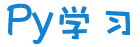注册    登录创作新主题

Python
 python开源   Django   Python   DjangoApp   pycharm
DATA
 docker   Elasticsearch

 问与答   闲聊   招聘   翻译   创业   分享发现   分享创造   求职   区块链   支付之战
aigc
 aigc   chatgpt
WEB开发
 linux   MongoDB   Redis   DATABASE   NGINX   其他Web框架   web工具   zookeeper   tornado   NoSql   Bootstrap   js   peewee   Git   bottle   IE   MQ   Jquery

Python88.com
 反馈   公告   社区推广

Py学习  »  机器学习算法

# 【深度学习】DN-DETR：引入去噪训练以加速收敛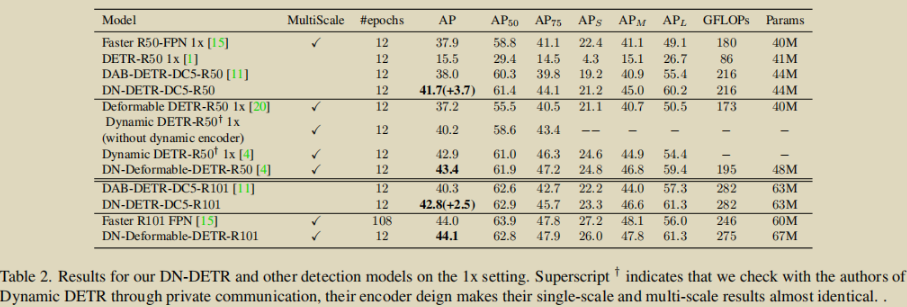12个 epochs 的性能对比“哦？作为 DETR 亮点之一的匈牙利匹配居然是慢收敛的凶手！？”炼丹界的群众们不禁惊呼。

Decoder queries 的学习

OK，我们已经“结合当前困局”& “更进一步”地理解了 decoder queries 的学习，接下来就该针对偏移量的学习来突破当前困境了！

DeNoising（DN）大法好

we leverage a denoising task as a training shortcut to make relative offset learning easier, as the denoising task bypasses bipartite matching.

feeds ground-truth bounding boxes with noises into Transformer decoder and trains the model to reconstruct the original boxes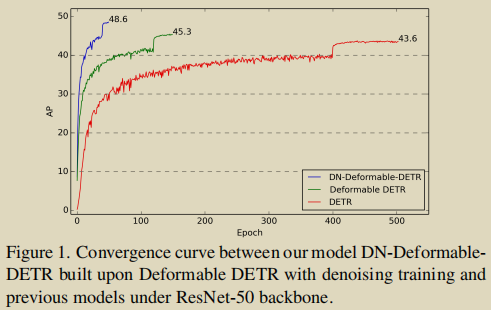OK，根据以上所述，我们知道，DN 任务的输入可以通过对 gt box 增加一些扰动（比如对 x、y、w、h 4 个分量都加上较小的值）来构造。但是，之前的工作为我们揭示了 decoder query 包含着 content & position 两部分，而现在这样就相当于只对 position 部分进行了加噪。

noised labes: 对 gt labels 加噪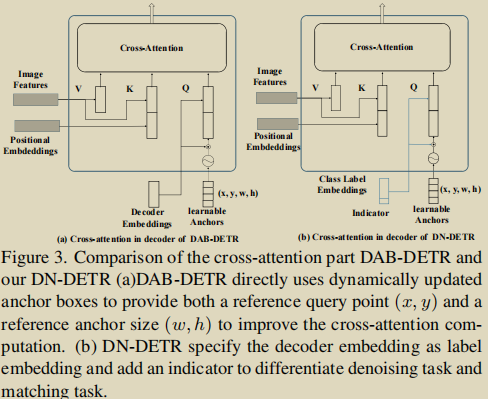noised boxes：对 gt boxes 加噪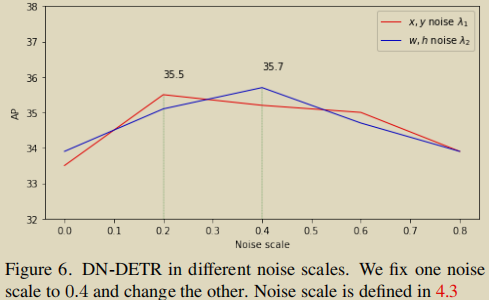dn groups:

CW 在前言里就提到了，DN 大法在模型训练时，没有破坏原来的匈牙利二分匹配 one-to-one 的逻辑，并且在推理时是去掉的，它只是在训练时打辅助 —— 帮助 DETR 更明确 query & gt 的对应关系。于是，在推理时，DN-DETR 输出的依然是基于匈牙利二分匹配的结果。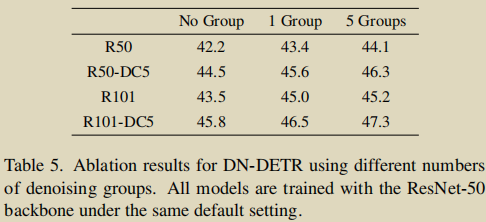• 匈牙利匹配任务的 queries 不能看到 DN任务的 queries

• DN 任务中，不同组的 queries 不能相互看到

• 其它情况均可见

noised labes, noised boxes & attention mask 做为 DN 大法的“三剑客”，作者对它们的 KPI 完成情况也进行了发布：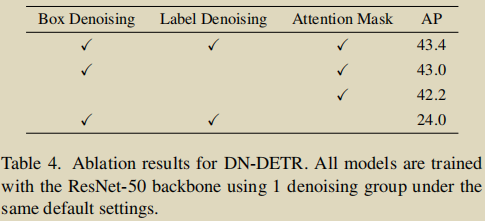IS（InStability）指标

DN-DETR 吹得头头是道，能够帮助 query & gt 的匹配更稳定、避免二义性等等，那么实际效果究竟如何？怎么去评估？又怎么量化呢？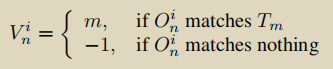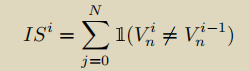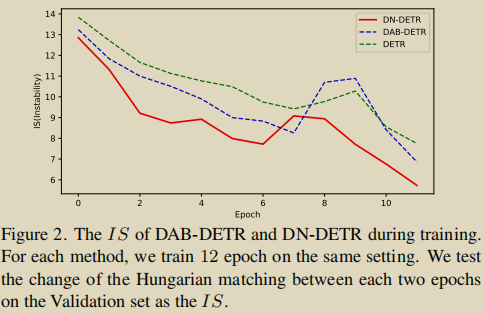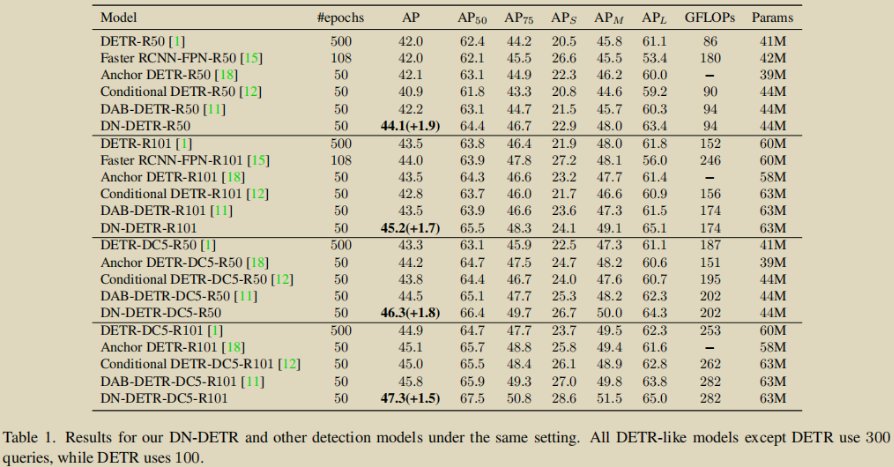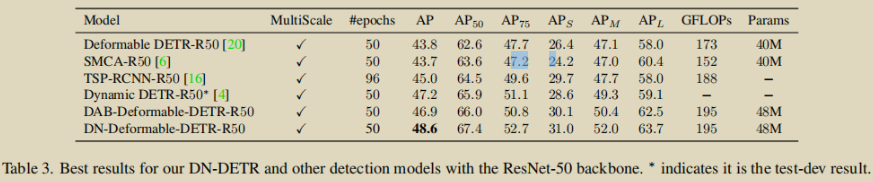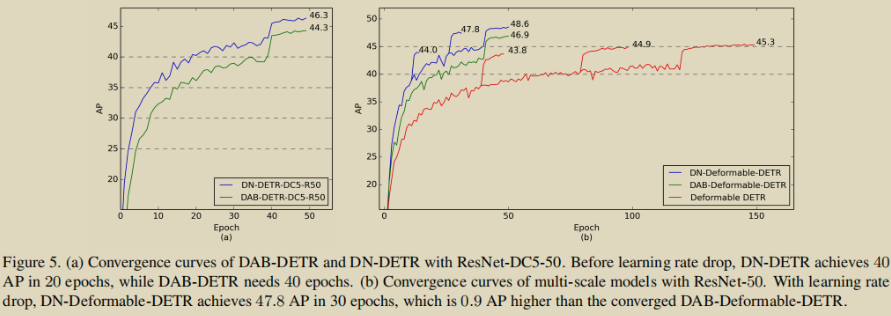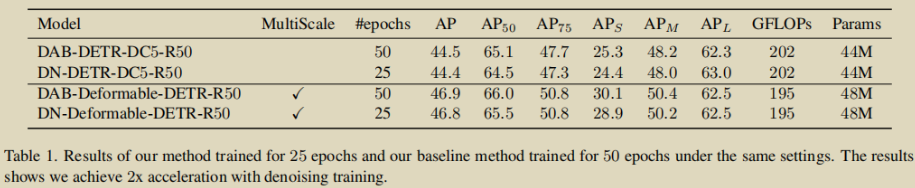（DAB-DETR: 老弟呀！本是同根生，相煎何太急...）

Re-thinking

CW 从代码实现中发现，DN task 的噪声是从均匀分布采样的，并且是在所有 dn groups 均匀采样，同时也缺乏对每个 dn group 有“针对性”的设置。这里有两个方向可以去探索。首先，如果使用其它分布的噪声会有怎样的效果(其实 paper 中也有提到)？第二，如何对不同的 group 做针对性设置，同时在每个 group 内保持相同的设置。

WOW~ 又到了最不无聊的部分了！

• 对原始 DETR queries 的改动

• 如何添加噪声 以及 分配去噪任务的标签

• 如何在 transformer 输入端兼容去噪任务与匈牙利匹配任务的 queries

• 如何在 transformer 输出端分离去噪任务与匈牙利匹配任务的输出结果

• 计算 loss 前需要对去噪任务的输出结果做哪些预处理

• 去噪任务的 loss（分类 loss & 回归 loss）

CW 再次强调哦，以下每节均是针对去噪任务而言，不涉及原始 DETR 的匈牙利匹配任务。

https://zhuanlan.zhihu.com/p/560513044

````def prepare_for_dn(dn_args, embedweight, batch_size, training, num_queries, num_classes, hidden_dim, label_enc):``    """``    prepare for dn components in forward function``    Args:``        dn_args: (targets, args.scalar, args.label_noise_scale,``                  args.box_noise_scale, args.num_patterns) from engine input``        embedweight: positional queries as anchor``        training: whether it is training or inference``        num_queries: number of queries``        num_classes: number of classes``        hidden_dim: transformer hidden dimenstion``        label_enc: label encoding embedding``    Returns: input_query_label, input_query_bbox, attn_mask, mask_dict``    """``    ``    if training:``        # targets 是 List[dict]，代表1個 batch 的標籤，其中每個 dict 是每張圖的標籤``        # scalar 代表的是 dn groups，去噪的組數，默認是 5``        targets, scalar, label_noise_scale, box_noise_scale, num_patterns = dn_args``    else:``        num_patterns = dn_args``    if num_patterns == 0:`

`        num_patterns = 1``    ''' 原始 DETR 匹配任务的 content & position queries '''``    # content 部分``    # 用於指示匹配(matching)任務的向量``    indicator0 = torch.zeros([num_queries * num_patterns, 1]).cuda()``    # label_enc 是 nn.Embedding()，其 weight 的 shape 是 (num_classes+1, hidden_dim-1)``    # 第一維之所以是 num_classes+1 是因為以下 tgt 的初始化值是 num_classes，因此要求 embedding 矩陣的第一維必須有 num_classes+1；``    # 而第二維之所以是 hidden_dim-1 是因為要留一個位置給以上的 indicator0``    # 由于去噪任务的 label noise 是在 gt label(0~num_classes-1) 上加噪，``    # 因此这里 tgt 的初始化值是 num_classes，代表 non-object，以区去噪任(dn)务和匹配(matching)任务``    # (hidden_dim-1,)->(num_queries*num_patterns,hidden_dim-1)``    tgt = label_enc(torch.tensor(num_classes).cuda()).repeat(num_queries * num_patterns, 1)``    # (num_queries*num_patterns,hidden_dim)``    tgt = torch.cat([tgt, indicator0], dim=1)``    # position 部分``    # (num_queries,4)->(num_query*num_patterns,4)``    refpoint_emb = embedweight.repeat(num_patterns, 1)````

```` ''' 训练期间，引入去噪任务相关的部分'''``    if training:``        ''' 计算一些索引，以便后续计算 loss 时用作 query & gt 的匹配 '''``        # list 中的每個都是值為 1 shape 為 (num_gt_img,) 的張量``        # 注意，每個張量的 shape 不一定一樣，因為每張圖片的 gt 數量不一定一致``        known = [(torch.ones_like(t['labels'])).cuda() for t in targets]``        # 该 batch 里每张图中各 gt 在圖片中的 index``        # torch.nonzero() 返回的是張量中值不為0的元素的索引，list 中的每個張量 shape 是 (num_gt_img,1)``        know_idx = [torch.nonzero(t) for t in known]``        # 该 batch 中各圖片的 gt 數量``        known_num = [sum(k) for k in known]````

````        # 对 gt 在整个 batch 中计算索引``        # (num_gts_batch,) 其中每個值都是1``        unmask_bbox = unmask_label = torch.cat(known)``        # (num_gts_batch,1)``        known_indice = torch.nonzero(unmask_label + unmask_bbox)``        # (num_gts_batch,)``        known_indice = known_indice.view(-1)``        # “复制”到所有去噪组``        # (num_gts_batch,)->(scalar,num_gts_batch)->(scalar*num_gts_batch)``        known_indice = known_indice.repeat(scalar, 1).view(-1)``        ''' 准备 gt labels & gt boxes '''``        # gt labels``        # (num_gts_batch,)``        labels = torch.cat([t['labels'] for t in targets])``        # gt boxes``        # (num_gts_batch,4)``        boxes = torch.cat([t['boxes'] for t in targets])``        # 每張圖片的 batch 索引，這個變量用於代表各圖片是第幾張圖``        # (num_gts_batch,)``        batch_idx = torch.cat([torch.full_like(t['labels'].long(), i) for i, t in enumerate(targets)])``        # 将以上“复制”到所有去噪组``        # (num_gts_batch,4)->(scalar*num_gts_batch,4)``        known_bboxs = boxes.repeat(scalar, 1)``        # (num_gts_batch,)->(scalar*num_gts_batch,)``        known_labels = labels.repeat(scalar, 1).view(-1)``        # (num_gts_batch,)->(scalar*num_gts_batch,)``        known_bid = batch_idx.repeat(scalar, 1).view(-1)``        # 用於在 gt labels上加噪``        known_labels_expaned = known_labels.clone()``        # 用於在 gt boxes 上加噪``        known_bbox_expand = known_bboxs.clone()````

``        ''' 对 gt labels 加噪 '''``        # noise on the label``        # label_noise_scale 是用於 gt classes 的噪聲概率，默認是 0.2，即有20%的噪聲比例``        if label_noise_scale > 0:``            # (scalar*num_gts_batch,) 從均勻分佈中採樣``            p = torch.rand_like(known_labels_expaned.float())``            # (scalar*num_gts_batch,)``            chosen_indice = torch.nonzero(p < (label_noise_scale)).view(-1)  # usually half of bbox noise``            # paper 中的 'flip' 操作，隨機選擇任意的類別作為噪聲類別``            new_label = torch.randint_like(chosen_indice, 0, num_classes)  # randomly put a new one here``            # 在 dim0 中使用 chosen_indice 作為 index，new_label 作為值``            known_labels_expaned.scatter_(0, chosen_indice, new_label)``        m = known_labels_expaned.long().to('cuda')``        # 加噪後的類別標籤對應的 embedding 向量``        # (scalar*num_gts_batch)->(scalar*num_gts_batch,hidden_dim-1)``        input_label_embed = label_enc(m)``        # 用於指示去噪(dn)任務的向量``        # (scalar*num_gts_batch,1)``        indicator1 = torch.ones([input_label_embed.shape, 1]).cuda()``        # 作为去噪任务的 content quries``        # add dn part indicator``        # (scalar*num_gts_batch,hidden_dim)``        input_label_embed = torch.cat([input_label_embed, indicator1], dim=1)``

````        ''' 对 gt boxes 加噪 '''``        # noise on the box````

# box_noise_scale 是用於 gt boxes 的 scale 超參(paper 中的 lambda)，默認是 0.4````        if box_noise_scale > 0:``            # 噪聲偏移量，作用在 gt boxes 上以實現中心點位移以及尺度縮放``            # (scalar*num_gts_batch,4)``            diff = torch.zeros_like(known_bbox_expand)``            # bbox 中心點坐標: w/2,h/2``            diff[:, :2] = known_bbox_expand[:, 2:] / 2``            # bbox 寬高: w,h``            diff[:, 2:] = known_bbox_expand[:, 2:]``            # 在原 gt boxes 上加上偏移量，并且保证加噪后框的中心点在原来的框内``            # torch.rand_like(known_bbox_expand) * 2 - 1.0 的值域是 [-1,1)``            known_bbox_expand += torch.mul((torch.rand_like(known_bbox_expand) * 2 - 1.0), diff).cuda() * box_noise_scale``            known_bbox_expand = known_bbox_expand.clamp(min=0.0, max=1.0)``        # 原 gt boxes 是 [0,1] 归一化的数值，于是这里进行反归一化``        # (scalar*num_gts_batch,4)``        input_bbox_embed = inverse_sigmoid(known_bbox_expand)````

queries 数量“对齐”到一致

````        ''' padding: 使得该 batch 中每張圖都擁有相同數量的 noised labels & noised boxes '''``        # 該 batch 中一張圖最多的 gt 數量``        single_pad = int(max(known_num))``        # 将以上“扩展”到所有去噪组``        pad_size = int(single_pad * scalar)``        padding_label = torch.zeros(pad_size, hidden_dim).cuda()``        padding_bbox = torch.zeros(pad_size, 4).cuda()``        ''' 將去噪(dn)任務和匹配(matching)任務的 queries 拼接在一起 '''``        # (batch_size,pad_size + num_queries*num_patterns,hidden_dim)````        input_query_label = torch.cat([padding_label, tgt], dim=0).repeat(batch_size, 1

, 1)````        # (batch_size,pad_size + num_queries*num_patterns,4)``        input_query_bbox = torch.cat([padding_bbox, refpoint_emb], dim=0).repeat(batch_size, 1, 1)``        ''' 由于以上 input_query_label & input_query_bbox 是 padded 的，``            因此要将每张图片真实有效的 noised lables(前面的 input_label_embed) & noised boxes(前面的 input_bbox_embed) 放到正确的位置上 '''``        # map in order``        map_known_indice = torch.tensor([]).to('cuda')``        if len(known_num):``            # 将 gt 在其所在圖片中排序，以计算索引``            # 以下 List 中每个 tensor 的值域是 [0,num_gt_img-1]``            # (num_gts_batch,)``            map_known_indice = torch.cat([torch.tensor(range(num)) for num in known_num])``            # 计算出去噪任务中真实有效的(非 padding 的) queries 对应的索引``            # 給每個去噪組加上一個對應的 offset，使得不同去噪組的 indices 可區分``            # i 的值域是 [0, scalar-1]，以上 map_known_indice 的值域是 [0,single_pad-1]，``            # 因此以下计算出的 map_known_indice 的值域不會超過 pad_size(即 single_pad * scalar)``            # (num_gts_batch*scalar,)``            map_known_indice = torch.cat([map_known_indice + single_pad * i for i in range(scalar)]).long()``        if len(known_bid):``            # 將去噪任务中真实有效的 noised lables & noised boxes “塞”到正确的位置上``            # known_pid 和 map_known_indice 的 shape 都是 (scalar*num_gts_batch)，一一對應``            input_query_label[(known_bid.long(), map_known_indice)] = input_label_embed``            input_query_bbox[(known_bid.long(), map_known_indice)] = input_bbox_embed````

attention mask 的设计在 DN-DETR 中很是关键，如果没有这一 part，那模型会成为通过作弊而拿到高分的坏家伙，并不能真正学到东西。

````        ''' 设置 attention mask 以防作弊 '''``        # 去噪任务 & 匹配任务 的 queries 总数``        tgt_size = pad_size + num_queries * num_patterns``        # (i,j) = True 代表 i 不可見 j``        attn_mask = torch.ones(tgt_size, tgt_size).to('cuda') < 0````

# match query cannot see the reconstruct````        # 令匹配任務的 queries 看不到做去噪任務的 queries，因為後者含有真實標籤的信息``        attn_mask[pad_size:, :pad_size] = True``        # reconstruct cannot see each other``        # 对于去噪任务的 queries，只有同组内的相互可见，避免跨组泄露真實標籤的信息，``        # 因为每组中，gt 和 query 是 one-to-one 的。``        # 于是，在同一组内，对于每个 query 来说，其它 queries 都不会有自己 gt 的信息``        for i in range(scalar):``            if i == 0:``                attn_mask[single_pad * i:single_pad * (i + 1), single_pad * (i + 1):pad_size] = True``            if i == scalar - 1:``                attn_mask[single_pad * i:single_pad * (i + 1), :single_pad * i] = True``            else:``                attn_mask[single_pad * i:single_pad * (i + 1), single_pad * (i + 1):pad_size] = True``                attn_mask[single_pad * i:single_pad * (i + 1), :single_pad * i] = True````

````        mask_dict = {``            'known_indice': torch.as_tensor(known_indice).long(),  # (scalar*num_gts_batch,) 每个 gt 在整个 batch 中的索引``            'batch_idx': torch.as_tensor(batch_idx).long(),  # (num_gts_batch,)  每个 gt 所在图片的 batch 索引``            'map_known_indice': torch.as_tensor(map_known_indice).long(),  # (num_gts_batch*scalar,)  噪声 queries(非 padding 的) 的索引``            'known_lbs_bboxes': (known_labels, known_bboxs),  # (scalar*num_gts_batch,), (scalar*num_gts_batch,4)``            'know_idx': know_idx,  # List[Tensor]: 其中每個 Tensor 的 shape 是 (num_gt_img,1)  每个 gt 在其图片中的索引``            'pad_size': pad_size  # 该 batch 中噪声 queries 的数量(包括 padding 的)``        }``    # 推理时仅有原始 DETR 匹配任务的 queries``    else:``        # (num_queries*num_patterns,hidden_dim)->(batch_size,num_queries*num_patterns,hidden_dim)``        input_query_label = tgt.repeat(batch_size, 1, 1)````        # (num_query*num_patterns,4)->(batch_size,num_query*num_patterns,4)

````        input_query_bbox = refpoint_emb.repeat(batch_size, 1, 1)``        attn_mask = None``        mask_dict = None``    # 將 batch 對應的維度置換到第二維(dim1)，以適配 transformer 的輸入``    # (num_queries,batch,hidden_dim)``    input_query_label = input_query_label.transpose(0, 1)``    # (num_queries,batch,4)``    input_query_bbox = input_query_bbox.transpose(0, 1)``    return input_query_label, input_query_bbox, attn_mask, mask_dict````

``def dn_post_process(outputs_class, outputs_coord, mask_dict):``    """``    post process of dn after output from the transformer``    put the dn part in the mask_dict``    """``    # 分離 去噪(dn)任務 和 原始 DETR 匹配(matching)任務 的預測結果``    if mask_dict and mask_dict['pad_size'] > 0:``        # 取出去噪任務的預測結果``        # (num_layers,batch,pad_size,num_classes)``        output_known_class = outputs_class[:, :, :mask_dict['pad_size'], :]``        # (num_layers,batch,pad_size,4)``        output_known_coord = outputs_coord[:, :, :mask_dict['pad_size'], :]``        # 將去噪任務的預測結果記錄到 mask_dict``        mask_dict['output_known_lbs_bboxes'] = (output_known_class, output_known_coord)``        # 讓 outputs_class & outputs_coord 保持為原始 DETR 匹配任務的預測結果，與原始 DETR 架構兼容``        outputs_class = outputs_class[:, :, mask_dict['pad_size']:, :]``        outputs_coord = outputs_coord[:, :, mask_dict['pad_size']:, :]``    return outputs_class, outputs_coord``

``def prepare_for_loss(mask_dict):``    """``    Prepare dn components to calculate loss``    Args:``        mask_dict: a dict that contains dn information``    """``    # (num_layers,batch,pad_size,num_classes), (num_layers,batch,pad_size,4)``    output_known_class, output_known_coord = mask_dict['output_known_lbs_bboxes']``    # (num_dn_groups*num_gts_batch,), (num_dn_groups*num_gts_batch,4)``    known_labels, known_bboxs = mask_dict['known_lbs_bboxes']``    # (num_dn_groups*num_gts_batch,) 非 padding 部分的 queries 索引``    map_known_indice = mask_dict['map_known_indice']``    # (num_dn_groups*num_gts_batch,) 將所有 gt 在 batch 中排序的索引``    known_indice = mask_dict['known_indice']``    # (num_gts_batch,) 每個 gt 所在圖片的 batch 索引(即是該 batch 中的第幾張圖)``    batch_idx = mask_dict['batch_idx']``    # (num_dn_groups*num_gts_batch,) 所有去噪組每個 gt/queries 所在圖片的 batch 索引``    bid = batch_idx[known_indice]``    # 過濾，僅保留非 padding 部分的 queries 對應的預測結果``    if len(output_known_class) > 0:``        # (num_layers,num_dn_groups*num_gts_batch,num_classes)``        output_known_class = output_known_class.permute(1, 2, 0, 3)[(bid, map_known_indice)].permute(1, 0, 2)``        # (num_layers,num_dn_groups*num_gts_batch,4)``        output_known_coord = output_known_coord.permute(1, 2, 0, 3)[(bid, map_known_indice)].permute(1, 0, 2)``    num_tgt = known_indice.numel()``    return known_labels, known_bboxs, output_known_class, output_known_coord, num_tgt``

dn loss

loss 会对 transformer 每层的输出都计算，整体逻辑封装在以下方法中：

````def compute_dn_loss(mask_dict, training, aux_num, focal_alpha):``    """``    Compute dn loss in criterion.``    Args:``        mask_dict: a dict for dn information``        training: training or inference flag``        aux_num: aux loss number``        focal_alpha: for focal loss``    """``    losses = {}``    # 先計算 transformer 最後一層預測結果對應的 loss``    if training and 'output_known_lbs_bboxes' in mask_dict:``        # 過濾掉 padding 部分的 queries 的預測結果，使得 gt 與 query 預測結果一一對應``        # labels & bboxs: (num_dn_groups*num_gts_batch,), (num_dn_groups*num_gts_batch,4)``        # output_class & output_coord: (num_layers,num_dn_groups*num_gts_batch,num_classes), (num_layers,num_dn_groups*num_gts_batch,4)``        known_labels, known_bboxs, output_known_class, output_known_coord, num_tgt = prepare_for_loss(mask_dict)``        # dn task 的分類損失：focal loss``        losses.update(tgt_loss_labels(output_known_class[-1], known_labels, num_tgt, focal_alpha))``        losses.update(tgt_loss_boxes(output_known_coord[-1], known_bboxs, num_tgt))``    else:``        losses['tgt_loss_bbox'] = torch.as_tensor(0.).to('cuda')``        losses['tgt_loss_giou'] = torch.as_tensor(0.).to('cuda')``        losses['tgt_loss_ce'] = torch.as_tensor(0.).to('cuda')``        losses['tgt_class_error'] = torch.as_tensor(0.).to('cuda')``    # 再計算 transformer 除最後一層外其餘每層預測結果對應的 loss``    if aux_num:``        for i in range(aux_num):``            # dn aux loss``            if training and 'output_known_lbs_bboxes' in mask_dict:``                l_dict = tgt_loss_labels(output_known_class[i], known_labels, num_tgt, focal_alpha)````

l_dict = {k + f'_{i}': v for k, v in l_dict.items()}````                losses.update(l_dict)``                l_dict = tgt_loss_boxes(output_known_coord[i], known_bboxs, num_tgt)``                l_dict = {k + f'_{i}': v for k, v in l_dict.items()}``                losses.update(l_dict)``            else:``                l_dict = dict()``                l_dict['tgt_loss_bbox'] = torch.as_tensor(0.).to('cuda')``                l_dict['tgt_class_error'] = torch.as_tensor(0.).to('cuda')``                l_dict['tgt_loss_giou'] = torch.as_tensor(0.).to('cuda')``                l_dict['tgt_loss_ce'] = torch.as_tensor(0.).to('cuda')``                l_dict = {k + f'_{i}': v for k, v in l_dict.items()}``                losses.update(l_dict)``    return losses````

````def tgt_loss_labels(src_logits_, tgt_labels_, num_tgt, focal_alpha, log=True):``    """Classification loss (NLL)``    targets dicts must contain the key "labels" containing a tensor of dim [nb_target_boxes]``    """``    if len(tgt_labels_) == 0:``        return {``            'tgt_loss_ce': torch.as_tensor(0.).to('cuda'),``            'tgt_class_error': torch.as_tensor(0.).to('cuda'),``        }``    # 增加 batch 維度``    # (num_dn_groups*num_gts_batch,num_classes)->(1,num_dn_groups*num_gts_batch,num_classes)``    # (num_dn_groups*num_gts_batch,)->(1,num_dn_groups*num_gts_batch)``    src_logits, tgt_labels = src_logits_.unsqueeze(0), tgt_labels_.unsqueeze(0)`

`    # 製作 one-hot 類別標籤``    # (1,num_dn_groups*num_gts_batch,num_classes+1)``    target_classes_onehot = torch.zeros([src_logits.shape, src_logits.shape, src_logits.shape + 1],``                                        dtype=src_logits.dtype, layout=src_logits.layout, device=src_logits.device)``    target_classes_onehot.scatter_(2, tgt_labels.unsqueeze(-1), 1)``    target_classes_onehot = target_classes_onehot[:, :, :-1]``    # 返回的 focal loss 是先在 src_logits.shape 求 mean，然後 sum()，最後除以 num_tgt，``    # 這裡乘以 src_logtis.shape(等於 num_tgt) 代表這個 loss_ce 是所有類別均攤到每個 query 的損失``    loss_ce = sigmoid_focal_loss(src_logits, target_classes_onehot, num_tgt, alpha=focal_alpha, gamma=2) * src_logits.shape``    losses = {'tgt_loss_ce': loss_ce}``    # accuracy 計算的是準確率(默認是 top1)，以百分比表示``    # 這個 tgt_class_error 則表示錯誤率，僅用作 log，不參與梯度計算``    losses['tgt_class_error'] = 100 - accuracy(src_logits_, tgt_labels_)``    return losses````

``def sigmoid_focal_loss(inputs, targets, num_boxes, alpha: float = 0.25, gamma: float = 2):``    """``    Loss used in RetinaNet for dense detection: https://arxiv.org/abs/1708.02002.``    Args:``        inputs: A float tensor of arbitrary shape.``                The predictions for each example.``        targets: A float tensor with the same shape as inputs. Stores the binary``                 classification label for each element in inputs``                (0 for the negative class and 1 for the positive class).``        alpha: (optional) Weighting factor in range (0,1) to balance``                positive vs negative examples. Default = -1 (no weighting).``        gamma: Exponent of the modulating factor (1 - p_t) to``               balance easy vs hard examples.``    Returns:``        Loss tensor``    """``    # 將原始 logit 轉換為 0~1 概率``    prob = inputs.sigmoid()``    # 計算二元交叉熵損失``    # (1,num_dn_groups*num_gts_batch,num_classes)``    ce_loss = F.binary_cross_entropy_with_logits(inputs, targets, reduction="none")``    # focal loss 的套路：降低置信度高的樣本(包括正負樣本)的權重，對原始 BCE loss 加權``    p_t = prob * targets + (1 - prob) * (1 - targets)``    loss = ce_loss * ((1 - p_t) ** gamma)``    # 對正負樣本加權``    if alpha >= 0:``        alpha_t = alpha * targets + (1 - alpha) * (1 - targets)``        loss = alpha_t * loss``    return loss.mean(1).sum() / num_boxes``

``def tgt_loss_boxes(src_boxes, tgt_boxes, num_tgt,):``    """Compute the losses related to the bounding boxes, the L1 regression loss and the GIoU loss``       targets dicts must contain the key "boxes" containing a tensor of dim [nb_target_boxes, 4]``       The target boxes are expected in format (center_x, center_y, w, h), normalized by the image size.``    """``    if len(tgt_boxes) == 0:``        return {``            'tgt_loss_bbox': torch.as_tensor(0.).to('cuda'),``            'tgt_loss_giou': torch.as_tensor(0.).to('cuda'),``        }``    ''' L1 loss '''``    # (num_dn_groups*num_gts_batch,4)``    loss_bbox = F.l1_loss(src_boxes, tgt_boxes, reduction='none')``    # losses = {}``    # losses['tgt_loss_bbox'] = loss_bbox.sum() / num_tgt``    # 所有回歸量(x,y,w,h)均攤到每個 query 的 loss(這裡的 num_tgt 是 num_dn_groups * num_gts_batch，代表所有去噪組 queries 的總數)``    losses = {'tgt_loss_bbox': loss_bbox.sum() / num_tgt}``    ''' GIoU loss '''``    loss_giou = 1 - torch.diag(``        # (n_src_boxes,n_tgt_boxes)``        box_ops.generalized_box_iou(``            box_ops.box_cxcywh_to_xyxy(src_boxes),``            box_ops.box_cxcywh_to_xyxy(tgt_boxes)``        )``    )``    losses['tgt_loss_giou'] = loss_giou.sum() / num_tgt``    return losses``

CW 觉得，DN-DETR 之所以能够加速，一方面是因为去噪任务中 query 与 gt 是确定性关系，而 DETR 的匈牙利匹配是一个动态匹配的过程，每个 query 在不同 epoch 可能会频繁切换对应的目标 gt。同时，这个去噪任务不依赖于 DETR 的匈牙利匹配，相当于接了条“捷径”，让模型知道每个 query 应该“拥抱”哪个 gt。

```